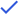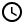# Using Google Sheets FILTER Function [w/ Single & Multiple Conditions]

Learn how to use FILTER on Google Sheets with single condition, multiple conditions, LARGE sort conditions, and SMALL sort conditions. Useful for data analysis and Google Sheets mastery.1. Use FILTER function with a single condition
2. Use FILTER function with multiple conditions, all need to be fulfilled
3. Use FILTER function with multiple conditions, at least need to be fulfilled
4. Use FILTER function to show the Top or Bottom 3 entries
5. The sample sheetChapter 1Chapter 2Chapter 3
1. Use FILTER function with a single condition
2. Use FILTER function with multiple conditions, all need to be fulfilled
3. Use FILTER function with multiple conditions, at least need to be fulfilled
4. Use FILTER function to show the Top or Bottom 3 entries
5. The sample sheetA simple function for filtering data with one or more conditions is FILTER. The FILTER function only requires you to specify the range of the data to be filtered and the conditions for filtering them.

## Use FILTER function with a single condition

The FILTER function follows the following syntax:

=FILTER(RANGE,CONDITION1,CONDITION2,...)

Where the RANGE is the range of the dataset that you want to filter and CONDITION1 and CONDITION2 are the conditions you want to use to filter the data.

At least one condition is required, and this is what we will show in this section.

For our example, we have the following data:

We want to filter the dataset to see who among them has an output score of more than 25. To do so, let us first specify the condition. The condition is written with the range of the column identified, then followed by a relation. For our example, we will write it as follows:

C2:C20>25

Since the entries in column C extends from C2 to C20. We will add this as the condition for our FILTER function:

=FILTER(A2:E20,C2:C20>25)

And the result looks like this:

In case that no entry fulfilled the conditions, the result would simply be #N/A.

Therefore, the steps for using FILTER with a single condition are easy:

Step 1. Enter FILTER function.

Step 2. Enter selected range as first argument (RANGE).

Step 3. Enter desired condition as second argument (CONDITION1).

## Use FILTER function with multiple conditions, all need to be fulfilled

As specified in the previous section, you can add more than one condition for the FILTER function to follow. The additional conditions have to be separated by a comma.

To improve upon our example, we want to add a condition to list down only entries whose quality score is more than 8.2. We can write the new function as follows:

=FILTER(A2:E20,C2:C20>25,D2:D20>8.2)

The result now looks like this:

Therefore, the steps for using FILTER with multiple conditions are similar to the ones above:

Step 1. Enter FILTER function.

Step 2. Enter selected range as first argument (RANGE).

Step 3. Enter desired condition as the next argument (CONDITION).

Step 4. Repeat step 3 for all of your conditions.

## Use FILTER function with multiple conditions, at least one need to be fulfilled

For this case, you can enclose two or more conditions using the OR operator (the plus sign). For our example, we will write the function as follows:

=FILTER(A2:E20,(C2:C20>25)+(D2:D20>8.2))

The plus sign between the two conditions tell the FILTER function to list down any entry that satisfies at least one of the given conditions.

The result now looks like this:

Therefore, here are the steps for using FILTER with multiple conditions, where at least one needs to be filled:

Step 1. Enter FILTER function.

Step 2. Enter selected range as first argument (RANGE).

Step 3. Enter desired condition as the second argument (CONDITION).

Step 4. In the same argument, add the plus symbol (+) and your other condition.

Step 5. Repeat step 4 as needed.

## Use FILTER function to show the Top or Bottom 3 entries

You can narrow down the results to list down only the top 3 entries or the bottom 3 entries by using the LARGE and SMALL functions.

Both the LARGE and SMALL functions list down the value of the nth ranking value. Their syntaxes are the following:

=LARGE(RANGE,NTH RANK)
=SMALL(RANGE,NTH RANK)

For example, if we run the following formula:

=LARGE(C2:C20,3)

It will list down the third highest value in the set.

Therefore, we will use the LARGE and SMALL functions to extract the nth highest or smallest value in the set, and use it as a reference for comparison.

To list down the top 3 entries for our first example, we will use the LARGE function as part of the condition:

=FILTER(A2:E20,C2:C20>=LARGE(C2:C20,3))

And the result looks like this:

You can do the same with the SMALL function, but be mindful of the relational operator you need to use:

=FILTER(A2:E20,C2:C20

So the result looks like this:

... so here comes the limitations of this technique. If more than one entry contains the same value, they will all be listed together in the list. Supposedly we list the bottom three entries here, but now six entries were listed. The best work arounds for this are using the Google Sheets Slicer or Filter View.

## The sample sheet

Here is our sample sheet that you can see how the FILTER function works:

Filter Function Sample Sheet
Oops! Something went wrong while submitting the form.

January 6, 2021

# Using Google Sheets FILTER Function [w/ Single & Multiple Conditions]A simple function for filtering data with one or more conditions is FILTER. The FILTER function only requires you to specify the range of the data to be filtered and the conditions for filtering them.

## Use FILTER function with a single condition

The FILTER function follows the following syntax:

=FILTER(RANGE,CONDITION1,CONDITION2,...)

Where the RANGE is the range of the dataset that you want to filter and CONDITION1 and CONDITION2 are the conditions you want to use to filter the data.

At least one condition is required, and this is what we will show in this section.

For our example, we have the following data:

We want to filter the dataset to see who among them has an output score of more than 25. To do so, let us first specify the condition. The condition is written with the range of the column identified, then followed by a relation. For our example, we will write it as follows:

C2:C20>25

Since the entries in column C extends from C2 to C20. We will add this as the condition for our FILTER function:

=FILTER(A2:E20,C2:C20>25)

And the result looks like this:

In case that no entry fulfilled the conditions, the result would simply be #N/A.

Therefore, the steps for using FILTER with a single condition are easy:

Step 1. Enter FILTER function.

Step 2. Enter selected range as first argument (RANGE).

Step 3. Enter desired condition as second argument (CONDITION1).

## Use FILTER function with multiple conditions, all need to be fulfilled

As specified in the previous section, you can add more than one condition for the FILTER function to follow. The additional conditions have to be separated by a comma.

To improve upon our example, we want to add a condition to list down only entries whose quality score is more than 8.2. We can write the new function as follows:

=FILTER(A2:E20,C2:C20>25,D2:D20>8.2)

The result now looks like this:

Therefore, the steps for using FILTER with multiple conditions are similar to the ones above:

Step 1. Enter FILTER function.

Step 2. Enter selected range as first argument (RANGE).

Step 3. Enter desired condition as the next argument (CONDITION).

Step 4. Repeat step 3 for all of your conditions.

## Use FILTER function with multiple conditions, at least one need to be fulfilled

For this case, you can enclose two or more conditions using the OR operator (the plus sign). For our example, we will write the function as follows:

=FILTER(A2:E20,(C2:C20>25)+(D2:D20>8.2))

The plus sign between the two conditions tell the FILTER function to list down any entry that satisfies at least one of the given conditions.

The result now looks like this:

Therefore, here are the steps for using FILTER with multiple conditions, where at least one needs to be filled:

Step 1. Enter FILTER function.

Step 2. Enter selected range as first argument (RANGE).

Step 3. Enter desired condition as the second argument (CONDITION).

Step 4. In the same argument, add the plus symbol (+) and your other condition.

Step 5. Repeat step 4 as needed.

## Use FILTER function to show the Top or Bottom 3 entries

You can narrow down the results to list down only the top 3 entries or the bottom 3 entries by using the LARGE and SMALL functions.

Both the LARGE and SMALL functions list down the value of the nth ranking value. Their syntaxes are the following:

=LARGE(RANGE,NTH RANK)
=SMALL(RANGE,NTH RANK)

For example, if we run the following formula:

=LARGE(C2:C20,3)

It will list down the third highest value in the set.

Therefore, we will use the LARGE and SMALL functions to extract the nth highest or smallest value in the set, and use it as a reference for comparison.

To list down the top 3 entries for our first example, we will use the LARGE function as part of the condition:

=FILTER(A2:E20,C2:C20>=LARGE(C2:C20,3))

And the result looks like this:

You can do the same with the SMALL function, but be mindful of the relational operator you need to use:

=FILTER(A2:E20,C2:C20

So the result looks like this: